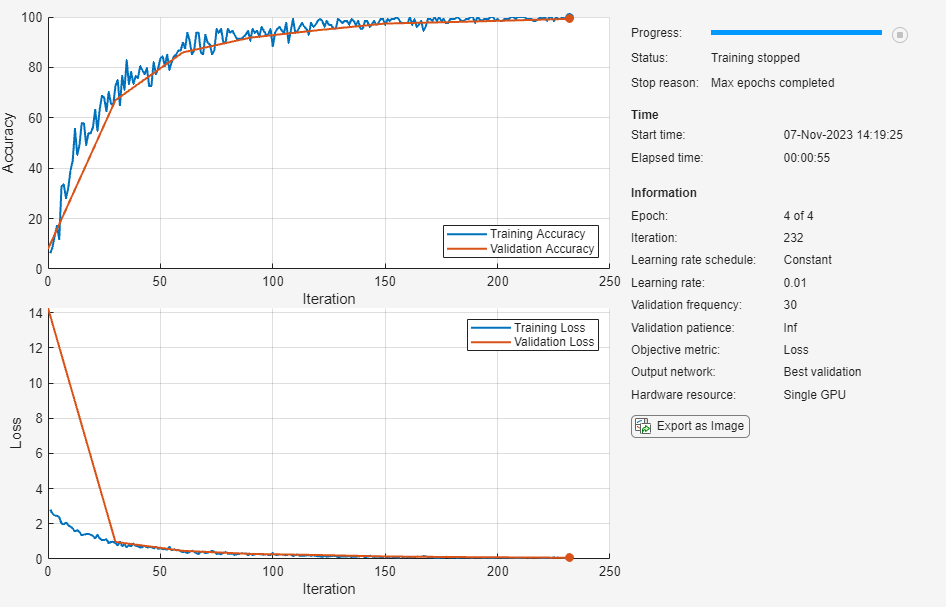# 创建简单的深度学习网络以用于分类

• 加载和浏览图像数据。

• 定义网络架构。

• 指定训练选项。

• 训练网络。

• 预测新数据的标签并计算分类准确度。

### 加载和浏览图像数据

```digitDatasetPath = fullfile(matlabroot,'toolbox','nnet','nndemos', ... 'nndatasets','DigitDataset'); imds = imageDatastore(digitDatasetPath, ... 'IncludeSubfolders',true,'LabelSource','foldernames');```

```figure; perm = randperm(10000,20); for i = 1:20 subplot(4,5,i); imshow(imds.Files{perm(i)}); end````labelCount = countEachLabel(imds)`
```labelCount=10×2 table Label Count _____ _____ 0 1000 1 1000 2 1000 3 1000 4 1000 5 1000 6 1000 7 1000 8 1000 9 1000 ```

```img = readimage(imds,1); size(img)```
```ans = 1×2 28 28 ```

### 指定训练集和验证集

```numTrainFiles = 750; [imdsTrain,imdsValidation] = splitEachLabel(imds,numTrainFiles,'randomize');```

### 定义网络架构

```layers = [ imageInputLayer([28 28 1]) convolution2dLayer(3,8,'Padding','same') batchNormalizationLayer reluLayer maxPooling2dLayer(2,'Stride',2) convolution2dLayer(3,16,'Padding','same') batchNormalizationLayer reluLayer maxPooling2dLayer(2,'Stride',2) convolution2dLayer(3,32,'Padding','same') batchNormalizationLayer reluLayer fullyConnectedLayer(10) softmaxLayer classificationLayer];```

ReLU 层批量归一化层后接一个非线性激活函数。最常见的激活函数是修正线性单元 (ReLU)。使用 `reluLayer` 创建 ReLU 层。

softmax 层 softmax 激活函数对全连接层的输出进行归一化。softmax 层的输出由总和为 1 的多个正数组成，这些数字随后可被分类层用作分类概率。使用 `softmaxLayer` 函数在最后一个全连接层后创建一个 softmax 层。

### 指定训练选项

```options = trainingOptions('sgdm', ... 'InitialLearnRate',0.01, ... 'MaxEpochs',4, ... 'Shuffle','every-epoch', ... 'ValidationData',imdsValidation, ... 'ValidationFrequency',30, ... 'Verbose',false, ... 'Plots','training-progress');```

### 使用训练数据训练网络

`net = trainNetwork(imdsTrain,layers,options);`### 对验证图像进行分类并计算准确度

```YPred = classify(net,imdsValidation); YValidation = imdsValidation.Labels; accuracy = sum(YPred == YValidation)/numel(YValidation)```
```accuracy = 0.9988 ```